• 本文首先介绍线性回归（具体概念解释可以参考CS229,吴恩达讲义的译文：传送门），包括其名称的由来python实现。在这之后引入了局部平滑技术，分析如何更好地你数据。接下来，本文将探讨回归在"欠拟合"情况下的...
之前博客中，我有介绍到分类机器学习算法，分类的目标变量是标称型数据，本篇博客将重点对连续型的数据做出预测及回归算法。有些人会问："回归能用来做什么？"其实回归能够做任何事情。
本文首先介绍线性回归（具体概念解释可以参考CS229,吴恩达讲义的译文：传送门），包括其名称的由来和python实现。在这之后引入了局部平滑技术，分析如何更好地你和数据。接下来，本文将探讨回归在"欠拟合"情况下的shrinkage技术，探讨偏差和方差的概念。
用线性回归来找到最佳拟合直线
在具体讲解线性回归之前，咱们先来看看从宏观上分析下线性回归。

线性回归  优点  结果易于理解，计算上不复杂  缺点  对非线性的数据拟合的不好  适用数据类型  数值型和标称型数据

说到回归，一般都是指线性回归，所以本文里的回归和线性回归代表同一个意思。需要说明的是，存在另一种称为非线性回归的回归模型，该模型输入与输出的关系与线性模型并不相同。
回归的步骤：

收集数据

准备数据：回归需要数值型数据，标称型数据将被转成二值型数据

分析数据：绘出数据的可视化二维图将有助于对数据做出理解和分析

训练数据：找到回归系数

测试算法：分析模型的效果（利用拟合系数corrcoef）

使用算法：使用回归，本质上是对分类方法的提升，因为这样可以预测来内需型数据而不仅仅是离散的类别标签

标准回归函数的实现：
回归系数w的求解公式如下：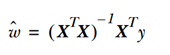需要注意的是上述公式中包含，也就是需要对矩阵求逆，因此需要在代码中对的逆矩阵是否存在做出判断。

from numpy import *
def standRegres(xArr,yArr):
xMat = mat(xArr); yMat = mat(yArr).T
xTx = xMat.T*xMat
if linalg.det(xTx) == 0.0:
print "This matrix is singular, cannot do inverse"
return
ws = xTx.I * (xMat.T*yMat)
return ws

1.2 局部加权线性回归（根据数据来局部调整预测）
线性回归的一个问题是有可能出现欠拟合现象，因为它求得是具有最小均方误差的无偏估计。所以有些方法允许在估计中引入一些偏差，从而降低预测的均方误差。其中的一个方法是局部加权线性回归（Locally Weighted Linear Regression , LWLR）。在该算法中，我们给待预测点附近的每个点赋予一定的权重，LWLR使用"核"来对附近的点赋予更高的权重。最常用的核就是高斯核，高斯核对应的权重如下：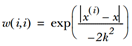这样就构建了一个只含对角元素的权重矩阵w，并且点x与x( i )越近,w(i,i)将会越大。上述公式包含一个需要用户指定的参数k，它决定了对附近的点赋予多大的权重，这也是使用LWLR时唯一需要考虑的参数下图显示了参数k与权重的关系。局部加权线性回归函数的实现：

def lwlr(testPoint,xArr,yArr,k=1.0):
xMat = mat(xArr); yMat = mat(yArr).T
m = shape(xMat)
weights = mat(eye((m)))
for j in range(m):                      #next 2 lines create weights matrix
diffMat = testPoint - xMat[j,:]     #
weights[j,j] = exp(diffMat*diffMat.T/(-2.0*k**2))
xTx = xMat.T * (weights * xMat)
if linalg.det(xTx) == 0.0:
print "This matrix is singular, cannot do inverse"
return
ws = xTx.I * (xMat.T * (weights * yMat))
return testPoint * ws

本文到此为止，介绍了提高预测精度的方法：局部加权回归，虽然局部加权回归比普通线性回归有更好的效果，但是由于每做一次预测，都必须使用整个数据集，所以会带来很大的计算量。接下来咱们介绍下另一种提高预测精度的方法，并分析它的优势所在。
1.3缩减系数来"理解"数据
如果数据的特征比样本点还多，会发现使用线性回归和之前的方法来做预测就会出错，因为如果特征比样本点还多（n>m）也就是说输入矩阵x不是满秩矩阵。非满秩矩阵在求逆时会出现问题。
为了解决这个问题，统计学家引入了岭回归（ridge regression）的概念，这就是本节将介绍的第一种缩减方法。接着是lasso法，该方法效果很好但计算复杂，本节最后介绍了第二种缩减方法，称为前向逐步回归，可以得到与lasso差不多的效果，且更容易实现。
岭回归
简单来说，岭回归就是在矩阵上加入一个lI从而使矩阵非奇异，进而能对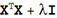求逆。其中矩阵I是一个m x m的单位矩阵，对角线上元素全为1，其他元素全为0。而l是一个用户定义的数值，在这种情况下，回归系数的计算公式将变成：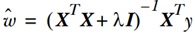岭回归最先用来处理特征多于样本数的情况，现在也用于在估计中加入偏差，从而得到更好的估计。这里通过引入l来限制了所有w之和，通过引入惩罚项，能够减少不重要的参数，这个技术在统计学中也叫做缩减（shrinkage）。
这里补充个小知识，大家知道岭回归的岭的由来么，岭回归使用了单位矩阵乘以常量l，我们观察其中的单位矩阵，可以看到值1贯穿整个对角线，其余元素全是0。形象地，在0构成的平面上有一条1组成的"岭"，这就是岭回归中的"岭"的由来。
缩减方法可以去掉不重要的参数，因此能更好地理解数据。此外，与简单的线性回归相比，缩减法能取得更好的预测效果。
岭回归代码

def ridgeRegres(xMat,yMat,lam=0.2):
xTx = xMat.T*xMat
denom = xTx + eye(shape(xMat))*lam
if linalg.det(denom) == 0.0:
print "This matrix is singular, cannot do inverse"
return
ws = denom.I * (xMat.T*yMat)
return ws

为了使用岭回归和缩减技术，首先需要对特征做标准化处理。具体做法就是所有的特征都减去各自的均值并除以方差。
还有一些其他的缩减方法，如lasso,LAR,PCA回归以及子集选择等。与岭回归一样，这些方法不敬可以提高预测精确率，而且可以解释回归系数。
Lasso
不难证明，在增加如下约束时，普通的最小二乘法回归会得到与岭回归的一样的公式：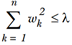上式限定了所有的回归系数平方和不能大于l。使用普通的最小二乘法回归在当两个或更多的特征相关时，可能会得出一个很大的正系数和很大的复习书。正是因为上述限制条件的存在，使用岭回归可以避免这个问题。
与岭回归类似，另一个缩减方法lasso也对回归系数做了限定，对应的约束条件如下：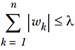为了在新的约束条件下解出回归系数，需要使用二次规划算法，这无疑极大地增加了计算复杂度，下面介绍一个更为简单的方法来得到结果，该方法叫做前向逐步回归。
前向逐步回归
前向逐步回归算法可以得到与lasso差不多的效果，但更简单。它属于一种贪心算法，即每一步都尽可能减少误差。一开始，所有的权重都设为1，然后每一步所做的决策是对某个权重增加或减少一个很小的值。
该算法伪代码：具体的实现算法如下：

def stageWise(xArr,yArr,eps=0.01,numIt=100):
xMat = mat(xArr); yMat=mat(yArr).T
yMean = mean(yMat,0)
yMat = yMat - yMean     #can also regularize ys but will get smaller coef
xMat = regularize(xMat)
m,n=shape(xMat)
#returnMat = zeros((numIt,n)) #testing code remove
ws = zeros((n,1)); wsTest = ws.copy(); wsMax = ws.copy()
for i in range(numIt):
print ws.T
lowestError = inf;
for j in range(n):
wsTest = ws.copy()
wsTest[j] += eps*sign
yTest = xMat*wsTest
wsMax = wsTest
ws = wsMax.copy()

接下来我们来探讨下偏差与方差的概念
权衡偏差与方差
在我们使用模型时，一旦发现模型和测量值存在差异，就说出现了误差。下图我们给出了训练误差与测试误差的曲线图，上面的曲线就是测试误差，下面的曲线就是训练误差。在前面的实验我们知道当核（k取值）越小，训练误差就会变小，下图，从左到右就表示了核逐渐减小的过程。一般认为，上述两个误差由三个部分组成：偏差、测量误差和随机噪声，前面我们通过引入三个越来越小的核来不断增大模型的方差。这样我们就可以通过实际效果来看偏差和方差间的折中效果。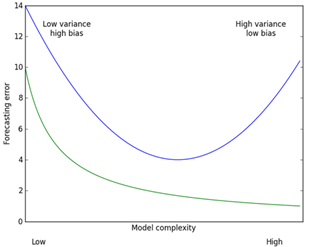展开全文机器学习
• 上次我们留了个两个问题没有仔细说明，个是局部加权线性回归，另个是岭回归。今天依次对这两种算法进行说明。、局部加权线性回归　欠拟合这种问题是仅仅凭借条直线来对数据点进行拟合的线性回归算法所无法...
　　上次我们留了个两个问题没有仔细说明，一个是局部加权线性回归，另一个是岭回归。今天依次对这两种算法进行说明。
一、局部加权线性回归
欠拟合这种问题是仅仅凭借一条直线来对数据点进行拟合的线性回归算法所无法避免的，而解决这种欠拟合问题的方法中，有一种最为简便，称之为局部加权线性回归。顾名思义，局部加权线性回归就是指给被预测点周围的数据点赋以不同的权重，让预测更加注重局部上的趋势而不是整体上的趋势，这样的操作一旦不注意就会造成过拟合，所以应用的时候要特别注意。  　　该算法对应的回归系数矩阵计算方法如下：

θ=(XTWX)−1XTWy
$\theta=(X^TWX)^{-1}X^TWy$
其中

W
$W$为权重矩阵，它是一个m∗m$m*m$的对角矩阵，训练集中的样本

xi
$x_i$所对应的权重存储在W(i,i)中。 为

n∗m
$n*m$的矩阵，

XTW
$X^TW$仍旧为

n∗m
$n*m$的矩阵，不同之处在于每个样本都被赋予了不同的权重。  　　权重的计算方式有很多种，其主要目标就是赋予待遇测点周围的数据点相对更高的权重，一般采用高斯核函数来实现这个目标，高斯核函数的形式如下：

W(i,i)=exp(|xi−x|−2k2)
$W(i,i)=exp( \frac{|x^i-x|}{-2k^2})$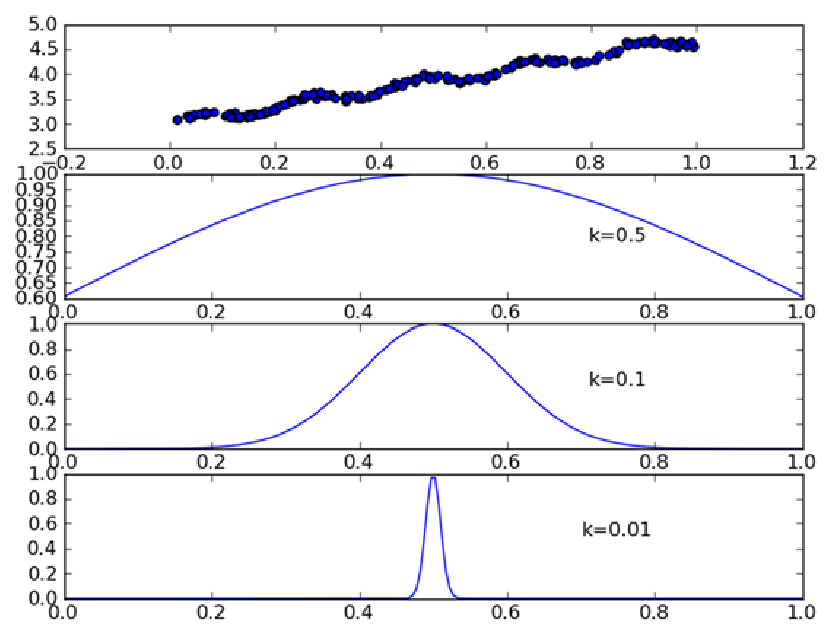我们可以注意到，

k
$k$的取值越小，权重衰减的越快，仅仅在要预测的点附近很有限的数据点才可以对回归系数的学习起到作用，其他的数据点权重为0无法参与到回归系数的学习过程当中。

这种方法有助于避免简单线性回归所带来的欠拟合的问题，然而它可能会带来过拟合的问题，如下图所示：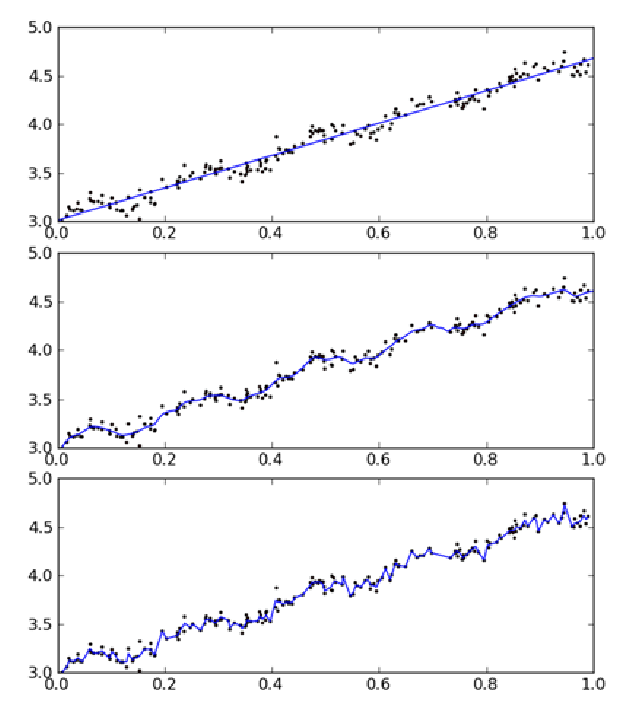从上图可以看出，中间的那张明显比剩下的两张更加合理一些，基本模拟出了数据的趋势，而图三就是k$k$值太小，出现了过拟合的情况，反而无法看出数据的规律。  　　除了容易出现过拟合的问题外，局部加权线性回归的计算量也相当大，因为它对每个待遇测点做预测时都必须遍历整个数据集才能得出回归系数向量，使用时要加以注意。
二、岭回归
使用线性回归的一个前提是矩阵

XTX
$X^TX$为满秩，这是因为若

XTX
$X^TX$ 奇异则无法求逆，式

θ=(XTX)−1XTy
$\theta=(X^TX)^{-1}X^Ty$ 无解。那么什么情况下矩阵

XTX
$X^TX$会出现无法求逆或者很难求逆的情况呢？当所有变量之间有较强的线性相关性时，或者变量之间的数据变化比较小或者部分变量之间有线性相关性时，矩阵

XTX
$X^TX$的行列式比较小，甚至趋近于0，这时矩阵

XTX
$X^TX$很难求逆，简单的线性回归方法无法适应这种情况。  　　在这种情况下，我们引入岭回归，回归系数的计算公式变为：

θ=(XTX+λI)−1XTy
$\theta=(X^TX+\lambda I)^{-1}X^Ty$  　　岭回归算法通过引入

λI
$\lambda I$来使得矩阵非奇异，可以求逆。岭回归中的“岭”就是指单位阵

I
$I$的对角线像一条山岭一样。不过这种表达还是不够直观，我们从原始的目标函数来看，岭回归的目标函数如下所示：
minw∑i=0n(yi−xTiθ)2+λ||θ||2min_w \sum_{i=0}^n(y_i-x_i^T\theta)^2+\lambda||\theta||^2  　　可以看到，岭回归是在简单线性回归的目标函数后面加了一个二范数惩罚项，其目的在于限制回归系数矩阵的取值，减少不必要的参数。显然，对于不同的

λ
$\lambda$，回归系数向量

θ
$\theta$会有不同的取值，反映

θ
$\theta$随

λ
$\lambda$变化的图像称为岭迹图，如下所示：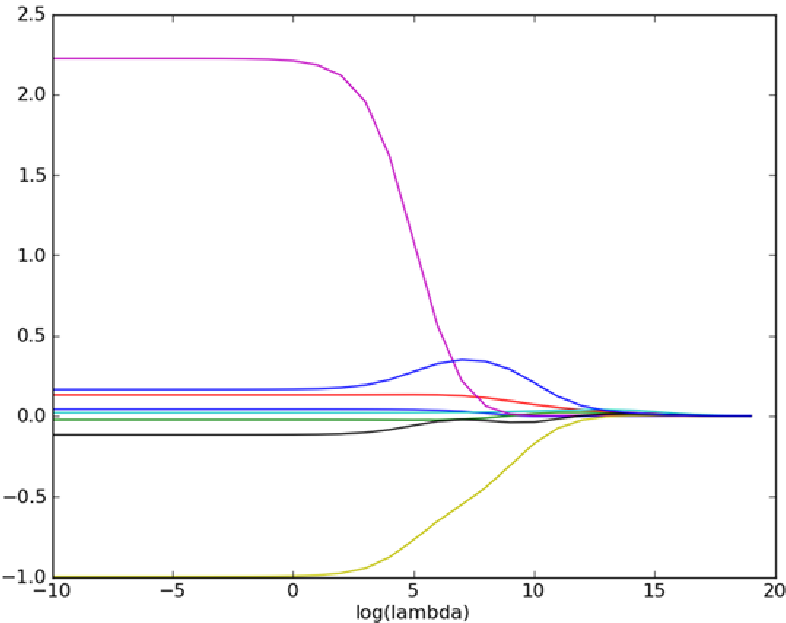可以看到当

λ
$\lambda$取值很小时，回归系数矩阵并没有什么变化，随着

λ
$\lambda$取值的逐渐增大，回归系数矩阵逐渐归于0。另外我们还可以根据岭迹图看出哪一个特征对最后的预测贡献最大（数据要归一化）。比如在上图中最上面的一条线，回归系数最大，对最后预测的贡献也就是最大。  简而言之，岭回归用于处理自变量之间高度相关的情形。线性回归的计算用的是最小二乘估计法，当自变量之间高度相关时，最小二乘回归估计的参数估计值会不稳定，这时如果在公式里加点东西，让它变得稳定，那就解决了这一问题了。岭回归就是这个思想。
展开全文算法 数据
• 机器学习算法的基本任务就是预测，预测目标按照数据类型可以分为两类：种是标称数据（通常表现为类标签），另种是连续数据（例如房价或者销售量等等）。针对标称数据的预测就是我们常说的分类，针对数值...
　　机器学习算法的基本任务就是预测，预测目标按照数据类型可以分为两类：一种是标称型数据（通常表现为类标签），另一种是连续型数据（例如房价或者销售量等等）。针对标称型数据的预测就是我们常说的分类，针对数值型数据的预测就是回归了。这里有一个特殊的算法需要注意，逻辑回归（logistic regression）是一种用来分类的算法，那为什么又叫“回归”呢？这是因为逻辑回归是通过拟合曲线来进行分类的。也就是说，逻辑回归只不过在拟合曲线的过程中采用了回归的思想，其本质上仍然是分类算法。  　　假如我们要预测某一地区的房价，我们可能会列出以下的式子进行估计：  　　  　　　　　　　　　　　　　　　　　　　房价 = 0.7 * 面积 + 0.19 * 房子的朝向  　　  　　 这个简单的式子就叫回归方程，其中0.7和0.19称为回归系数，面积和房子的朝向称为特征。有了这些概念，我们就可以说，回归实际上就是求回归系数的过程。在这里我们看到，房价和面积以及房子的朝向这两个特征呈线性关系，这种情况我们称之为线性回归。当然还存在非线性回归，在这种情况下会考虑特征之间出现非线性操作的可能性（比如相乘或者相除），由于情况有点复杂，不在这篇文章的讨论范围之内。  　　简便起见，我们规定代表输入数据的矩阵为

X
$\mathbf X$ （维度为m*n，m为样本数，n为特征维度），回归系数向量为

θ
$\theta$（维度为n*1）。对于给定的数据矩阵

X
$\mathbf X$ ，其预测结果由：

Y=Xθ
$\mathbf Y= \mathbf X \theta$ 这个式子给出。我们手里有一些现成的x和y作为训练集，那么如何根据训练集找到合适的回归系数向量

θ
$\theta$是我们要考虑的首要问题，一旦找到

θ
$\theta$，预测问题就迎刃而解了。在实际应用中，我们通常认为能带来最小平方误差的

θ
$\theta$就是我们所要寻找的回归系数向量。平方误差指的是预测值与真实值的差的平方。采用平方这种形式的目的在于规避正负误差的互相抵消。所以，我们的目标函数如下所示：

minθ∑i=0m(yi−xTiθ)2

min_\theta\sum_{i=0}^m  (y_i-x_i^T\theta)^2  　　这里的m代表训练样本的总数。对这个函数的求解有很多方法，由于网络上对于详细解法的相关资料太少，下面展示一种利用正规方程组的解法：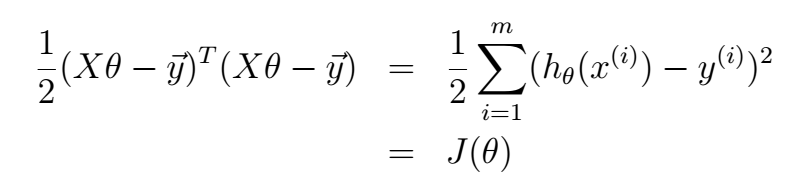(1)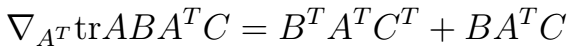∇AtrAB=BT
$\nabla_AtrAB=B^T$(2)  　　针对上式不太清楚的朋友可以看我之前写的这篇博文：http://blog.csdn.net/qrlhl/article/details/47758509。根据以上式子，解法如下：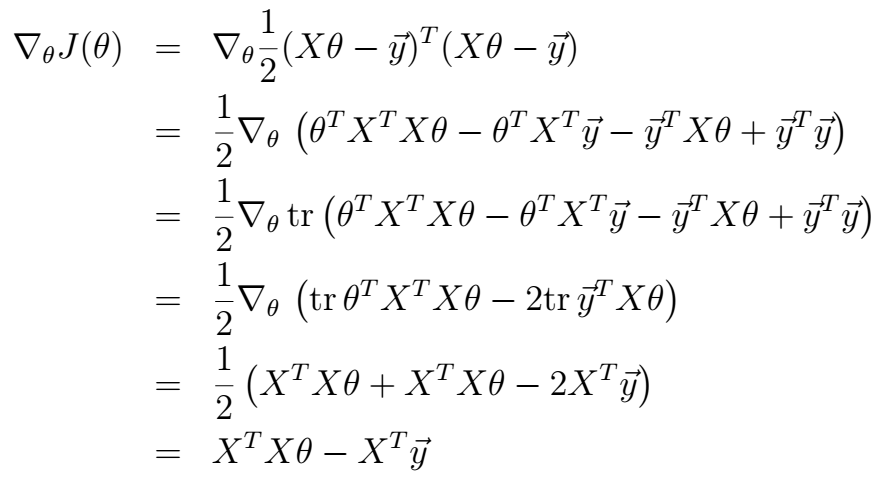令其等于0，即可得：

θ=(XTX)−1XTy
$\theta = (X^TX)^{-1}X^Ty$ 。有一些需要说明的地方：第三步是根据实数的迹和等于本身这一事实推导出的（括号中的每一项都为实数），第四步是根据式（2）推导出来的。第五步是根据式（1）推导出来的，其中的C为单位矩阵

I
$I$。这样，我们就得到了根据训练集求得回归系数矩阵θ$\theta$的方程。这种方法的特点是简明易懂，不过缺点也很明显，就是

XTX
$X^TX$ 这一项不一定可以顺利的求逆。由于只有满秩才可以求逆，这对数据矩阵

X
$X$提出了一定的要求。有人也许会问XTX$X^TX$不是满秩的情况下怎么办？这个时候就要用到岭回归（ridge regression）了，这一部分留到下次再讲。  　　说了这么久，放一张图上来看看线性回归的效果：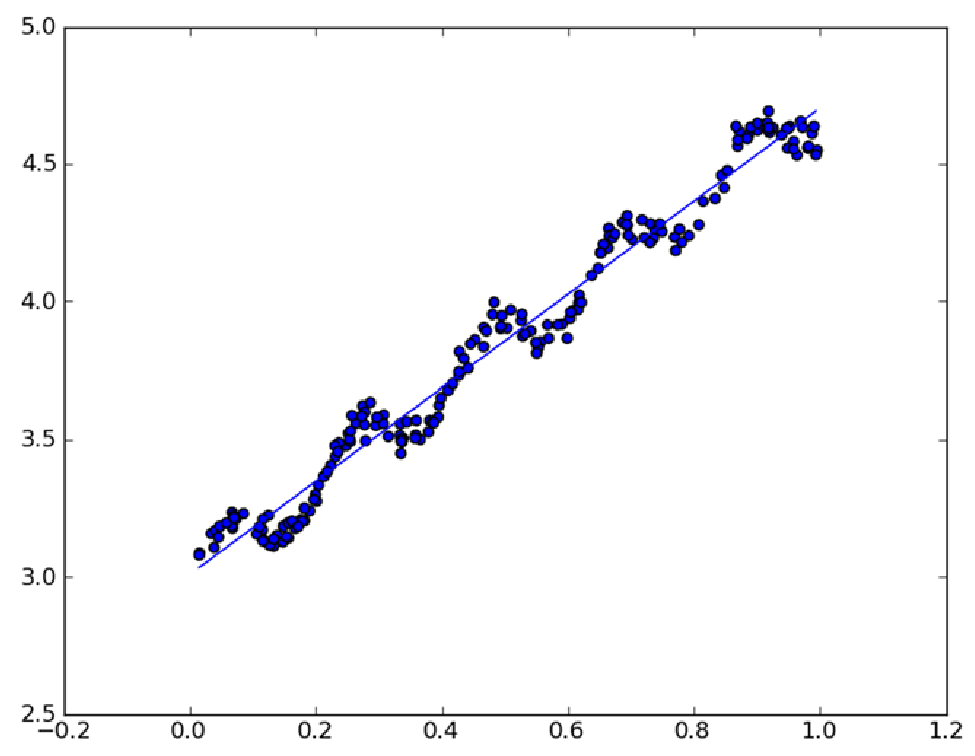可以看到，直线较好的拟合了数据点的变化趋势，可以作出相对较好的预测。但是细心的朋友可能会发现，如果在数据点的每一段用一小段直线拟合也许会获得更加好的结果，就像这样：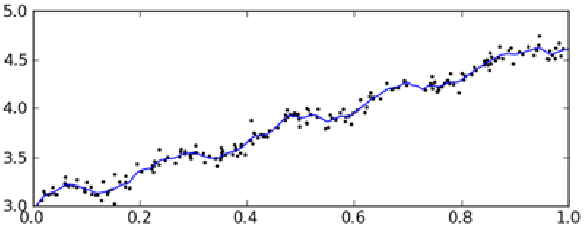这种方法叫做局部加权线性回归（Locally Weighted Linear Regression，LWLR），关于这种方法的细节，我们下次再说。
展开全文数据 机器学习
• 线性回归作为回归分析技术，其分析的因变量属于连续变量，如果因变量转变为离散变量，将转换为分类问题。回归分析属于有监督学习问题，本博客将重点回顾标准线性回归知识点，并就线性回归中可能出现的问题...

线性回归作为一种回归分析技术，其分析的因变量属于连续型变量，如果因变量转变为离散型变量，将转换为分类问题。回归分析属于有监督学习问题，本博客将重点回顾标准线性回归知识点，并就线性回归中可能出现的问题进行简单探讨，引出线性回归的两个变种岭回归以及Lasso回归，最后通过sklearn库模拟整个回归过程。

目录结构

线性回归的一般形式线性回归中可能遇到的问题过拟合问题及其解决方法线性回归代码实现岭回归与Lasso回归岭回归以及Lasso回归代码实现
线性回归的一般形式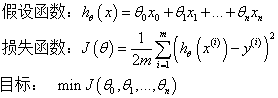线性回归中可能遇到的问题
求解损失函数的最小值有两种方法：梯度下降法以及正规方程，两者的对比在附加笔记中有列出。特征缩放：即对特征数据进行归一化操作，进行特征缩放的好处有两点，一是能够提升模型的收敛速度，因为如果特征间的数据相差级别较大的话，以两个特征为例，以这两个特征为横纵坐标绘制等高线图，绘制出来是扁平状的椭圆，这时候通过梯度下降法寻找梯度方向最终将走垂直于等高线的之字形路线，迭代速度变慢。但是如果对特征进行归一化操作之后，整个等高线图将呈现圆形，梯度的方向是指向圆心的，迭代速度远远大于前者。二是能够提升模型精度。学习率α的选取：如果学习率α选取过小，会导致迭代次数变多，收敛速度变慢；学习率α选取过大，有可能会跳过最优解，最终导致根本无法收敛。

过拟合问题及其解决方法
问题：以下面一张图片展示过拟合问题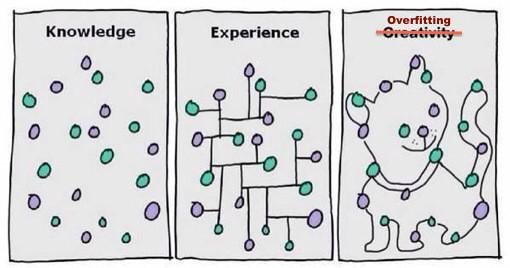解决方法：(1)：丢弃一些对我们最终预测结果影响不大的特征，具体哪些特征需要丢弃可以通过PCA算法来实现；(2)：使用正则化技术，保留所有特征，但是减少特征前面的参数θ的大小，具体就是修改线性回归中的损失函数形式即可，岭回归以及Lasso回归就是这么做的。
线性回归代码示例
import matplotlib.pyplot as plt
import numpy as np
from sklearn import datasets, linear_model, discriminant_analysis, cross_validation

return cross_validation.train_test_split(diabetes.data, diabetes.target, test_size=0.25, random_state=0)

def test_LinearRegression(*data):
X_train, X_test, y_train, y_test = data
#通过sklearn的linear_model创建线性回归对象
linearRegression = linear_model.LinearRegression()
#进行训练
linearRegression.fit(X_train, y_train)
#通过LinearRegression的coef_属性获得权重向量,intercept_获得b的值
print("权重向量:%s, b的值为:%.2f" % (linearRegression.coef_, linearRegression.intercept_))
#计算出损失函数的值
print("损失函数的值: %.2f" % np.mean((linearRegression.predict(X_test) - y_test) ** 2))
#计算预测性能得分
print("预测性能得分: %.2f" % linearRegression.score(X_test, y_test))

if __name__ == '__main__':
#获得数据集
X_train, X_test, y_train, y_test = load_data()
#进行训练并且输出预测结果
test_LinearRegression(X_train, X_test, y_train, y_test)
线性回归示例输出
权重向量:[ -43.26774487 -208.67053951  593.39797213  302.89814903 -560.27689824
261.47657106   -8.83343952  135.93715156  703.22658427   28.34844354], b的值为:153.07
损失函数的值: 3180.20
预测性能得分: 0.36

岭回归与Lasso回归

岭回归与Lasso回归的出现是为了解决线性回归出现的过拟合以及在通过正规方程方法求解θ的过程中出现的x转置乘以x不可逆这两类问题的，这两种回归均通过在损失函数中引入正则化项来达到目的，具体三者的损失函数对比见下图：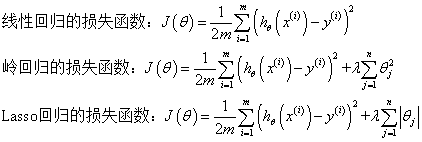其中λ称为正则化参数，如果λ选取过大，会把所有参数θ均最小化，造成欠拟合，如果λ选取过小，会导致对过拟合问题解决不当，因此λ的选取是一个技术活。  岭回归与Lasso回归最大的区别在于岭回归引入的是L2范数惩罚项，Lasso回归引入的是L1范数惩罚项，Lasso回归能够使得损失函数中的许多θ均变成0，这点要优于岭回归，因为岭回归是要所有的θ均存在的，这样计算量Lasso回归将远远小于岭回归。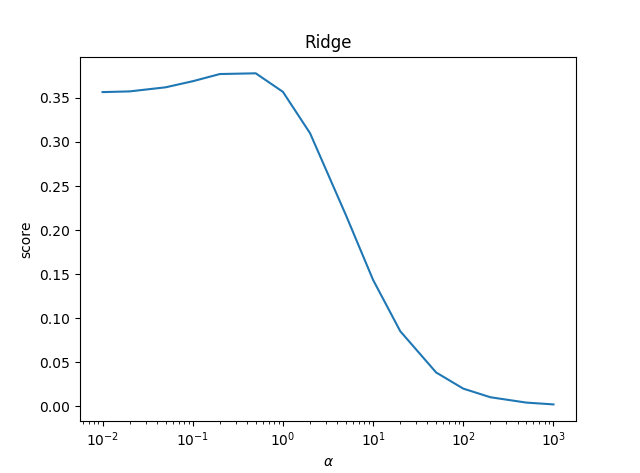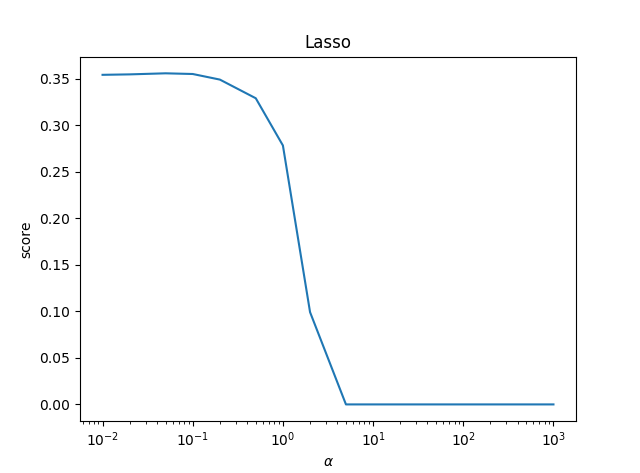可以看到，Lasso回归最终会趋于一条直线，原因就在于好多θ值已经均为0，而岭回归却有一定平滑度，因为所有的θ值均存在。

岭回归以及Lasso回归代码实现

岭回归代码示例
import matplotlib.pyplot as plt
import numpy as np
from sklearn import datasets, linear_model, discriminant_analysis, cross_validation

return cross_validation.train_test_split(diabetes.data, diabetes.target, test_size=0.25, random_state=0)

def test_ridge(*data):
X_train, X_test, y_train, y_test = data
ridgeRegression = linear_model.Ridge()
ridgeRegression.fit(X_train, y_train)
print("权重向量:%s, b的值为:%.2f" % (ridgeRegression.coef_, ridgeRegression.intercept_))
print("损失函数的值:%.2f" % np.mean((ridgeRegression.predict(X_test) - y_test) ** 2))
print("预测性能得分: %.2f" % ridgeRegression.score(X_test, y_test))

#测试不同的α值对预测性能的影响
def test_ridge_alpha(*data):
X_train, X_test, y_train, y_test = data
alphas = [0.01, 0.02, 0.05, 0.1, 0.2, 0.5, 1, 2, 5, 10, 20, 50, 100, 200, 500, 1000]
scores = []
for i, alpha in enumerate(alphas):
ridgeRegression = linear_model.Ridge(alpha=alpha)
ridgeRegression.fit(X_train, y_train)
scores.append(ridgeRegression.score(X_test, y_test))
return alphas, scores

def show_plot(alphas, scores):
figure = plt.figure()
ax.plot(alphas, scores)
ax.set_xlabel(r"$\alpha$")
ax.set_ylabel(r"score")
ax.set_xscale("log")
ax.set_title("Ridge")
plt.show()

if __name__ == '__main__':
#使用默认的alpha
#获得数据集
#X_train, X_test, y_train, y_test = load_data()
#进行训练并且预测结果
#test_ridge(X_train, X_test, y_train, y_test)

#使用自己设置的alpha
X_train, X_test, y_train, y_test = load_data()
alphas, scores = test_ridge_alpha(X_train, X_test, y_train, y_test)
show_plot(alphas, scores)

Lasso回归代码示例
import matplotlib.pyplot as plt
import numpy as np
from sklearn import datasets, linear_model, discriminant_analysis, cross_validation

return cross_validation.train_test_split(diabetes.data, diabetes.target, test_size=0.25, random_state=0)

def test_lasso(*data):
X_train, X_test, y_train, y_test = data
lassoRegression = linear_model.Lasso()
lassoRegression.fit(X_train, y_train)
print("权重向量:%s, b的值为:%.2f" % (lassoRegression.coef_, lassoRegression.intercept_))
print("损失函数的值:%.2f" % np.mean((lassoRegression.predict(X_test) - y_test) ** 2))
print("预测性能得分: %.2f" % lassoRegression.score(X_test, y_test))

#测试不同的α值对预测性能的影响
def test_lasso_alpha(*data):
X_train, X_test, y_train, y_test = data
alphas = [0.01, 0.02, 0.05, 0.1, 0.2, 0.5, 1, 2, 5, 10, 20, 50, 100, 200, 500, 1000]
scores = []
for i, alpha in enumerate(alphas):
lassoRegression = linear_model.Lasso(alpha=alpha)
lassoRegression.fit(X_train, y_train)
scores.append(lassoRegression.score(X_test, y_test))
return alphas, scores

def show_plot(alphas, scores):
figure = plt.figure()
ax.plot(alphas, scores)
ax.set_xlabel(r"$\alpha$")
ax.set_ylabel(r"score")
ax.set_xscale("log")
ax.set_title("Ridge")
plt.show()

if __name__=='__main__':
X_train, X_test, y_train, y_test = load_data()
# 使用默认的alpha
#test_lasso(X_train, X_test, y_train, y_test)
# 使用自己设置的alpha
alphas, scores = test_lasso_alpha(X_train, X_test, y_train, y_test)
show_plot(alphas, scores)

附上学习笔记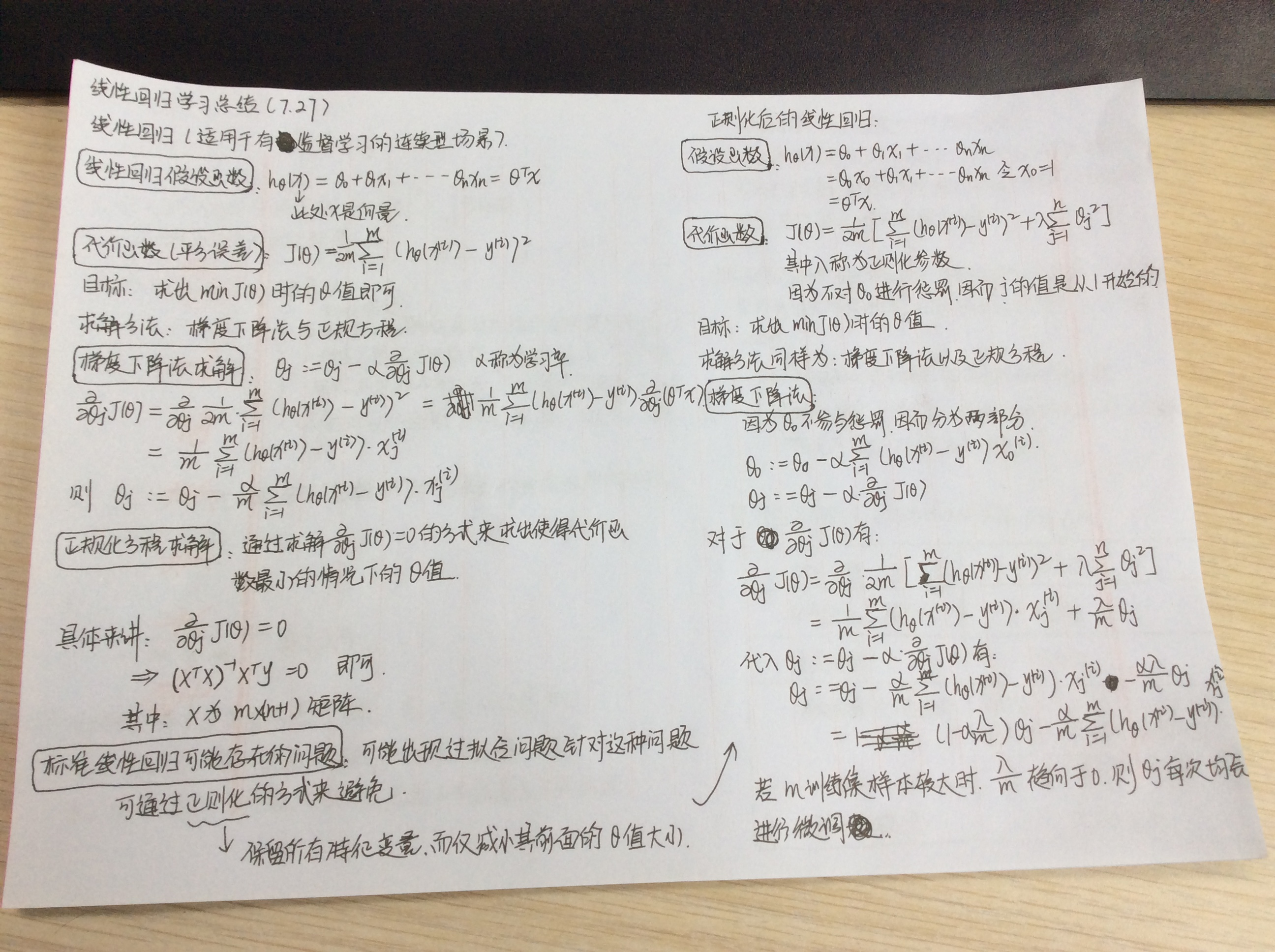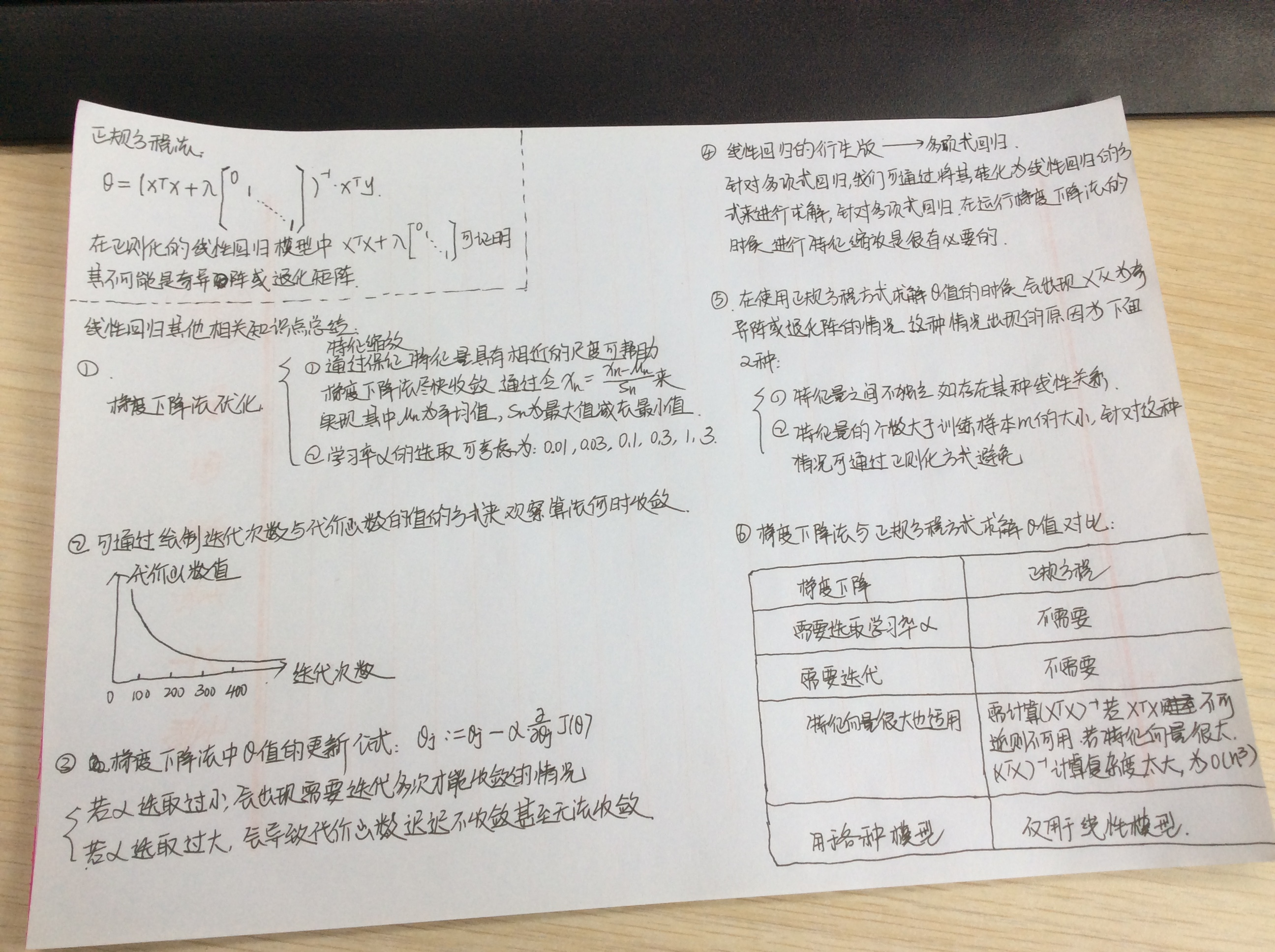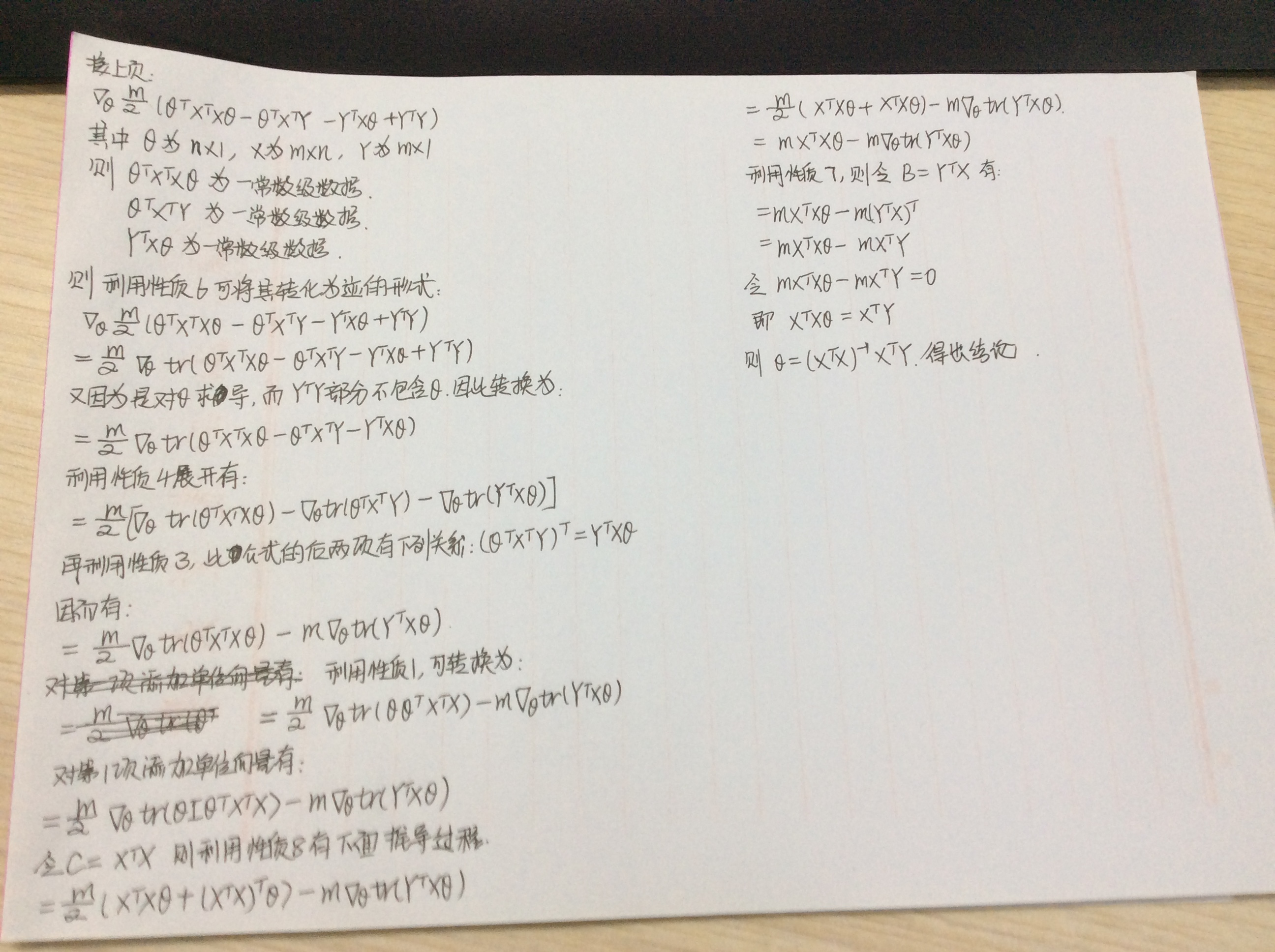参考文献
python大战机器学习Andrew Ng机器学习公开课http://www.jianshu.com/p/35e67c9e4cbfhttp://freemind.pluskid.org/machine-learning/sparsity-and-some-basics-of-l1-regularization/#ed61992b37932e208ae114be75e42a3e6dc34cb3
展开全文机器学习
• 对离散型和连续特征列的选择 、CART分类树（待预测结果为离散数据）： 选择具有最小Gain_GINI的属性及其属性值，作为最优分裂属性以及最优分裂属性值。Gain_GINI值越小，说明分之后的子样本的“纯净度”越高...
• 逻辑回归模型一般用来解决分类问题。 Binary Classification 一般的分类问题，输出 yyy 只有 {0, 1} 两个离散值，也有 {-1, 1} 的情况。 以个图像识别问题为例，判断图片中是否有猫存在，0...分类问题
• 叫做GWR，但是这个软件暂时我还没有用过，所以等我先学习一下，把他放到最后才说了，先用比较熟悉的，比如ArcGIS、比如R语言，这些来讲讲（还有个我非常熟悉的软件是GEODA，可惜GEODA仅支持回归分析，不支持地理...空间统计 ArcGIS
• 利用光学仿真软件TracePro对具有3种不同折射率(1.93、2.002.20)的回归反射器件结构单元(玻璃微珠)的回归反射性能进行模拟分析,得到了光线垂直入射时采用普通基底的传统开放型回归器件结构单元的理论反射率仅为23.6...
• 回归预测数值数据.回归分析1.概念回归分析是种数学模型。当因变量自变量为线性关系时，它是种特殊的线性模型。  最简单的情形是一元线性回归，由大体上有线性关系的个自变量和一个因变量组成；模型...
• 【机器学习】《机器学习实战》读书笔记及代码 总目录 ... ————————————————...目录本章内容1、回归2、用线性回归找到最佳拟合直线3、局部加权线性回归4、示例：预测鲍鱼的年龄5、缩减系数来“理解”...机器学习实战 机器学习 学习笔记
• 前四节主要讲述了回归问题，回归属于有监督学习中的种方法。该方法的核心思想是从连续统计数据中得到数学模型，然后将该数学模型用于预测或者分类。该方法处理的数据可以是多维的。  讲义最初介绍了个基本...training function 算法 扩展
• （2）准备数据：回归需要数值数据，标称数据将被转化成数据 （3）分析数据：绘出数据的可视化维图将有助于对数据做出理解分析，在采用缩减法求得新回归系数之后，可以将新拟合线在图上作为对比 （4）...python 机器学习 Regression
• 在此基础上，通过构建次规划模型对函数非参数回归预测模型的预测曲线进行修正，使其满足待预测日负荷特性指标要求。利用某省级电网夏季典型日负荷数据美国PJM电力公司冬季典型日负荷数据对所提方法进行测试，...
• 主要分析数值自变量与数值自变量之间的关系。 从变量个数上看，可分为简单相关与简单回归分析多元相关与多元回归分析；从变量之间的关系形态上看，有线性相关与线性回归分析非线性相关与非线性回归分析。1 ...
• ## 二值逻辑回归

千次阅读 2018-08-07 15:26:20
值逻辑回归 　在许多社会经济问题中，所研究的因变量往往只有两个可能的结果，这样的结果可以用虚拟变量来表示，虚拟变量的取值可取01。 　针对０－１的因变量，我们对回归模型应该做下面的改进。 　首先...机器学习
• 本篇内容本来想在写在上篇博客中的，那样篇幅过长，就单独提...本篇文章采用两种方式实现线性回归种是使用scikit-learn。而通过上篇博客，我们已经知道了最小二乘法求解线性回归参数，所以完全可以自己手动实现。机器学习 最小二乘
• ## 回归

千次阅读 2016-11-15 12:32:43
什么是回归回归实际上就是“最佳拟合”。  根据已有的数据拟合出条最佳的直线、曲线、超平面或函数等，用于预测其它数据的目标值。... 回归的目的就是预测数值的目标值。  下面介绍几种常见的回归方式。机器学习算法
• Probit回归： Probit回归全称probability unit，翻译过来叫做概率单位法，蛮拗口的个名字。...Probit回归经常拿来logistic回归作比较，通常对于分类变量来说，这两个回归计算出来的概率是非常相似的。（虽然log
• 回归模型初步建立起了自变量因变量之间的关系，这个关系式包括两部分，部分是自变量的线性函数部分，另部分是剩余误差项 ；回归函数，是描述随机变量η的平均值即期望是如何依赖于自变量x的函数；回归方程它是...
• 对非齐次等式约束线性回归模型提出种有偏估计，即条件岭估计，证明了在一定的条件下，在均方误差及均方误差矩阵意义下都优于回归系数的约束最小二乘估计，并给出了两次随机数据模拟的结果，模拟数据结果表明在...
• 线性回归模型 线性函数的定义如下： h(x)=w1x1+w2x2+...+wdxd+b=wTx+bh(\bm{x})=w_{1}x_{1}+w_{2}x_{2}+...+w_{d}x_{d}+b=\bm{w}^{T}\bm{x}+bh(x)=w1​x1​+w2​x2​+...+wd​xd​+b=wTx+b 给定数据集D={(xi,yi)}...
• 具体点说，在回归中，假定因变量Y自变量X之间的模型，然后计算模型中的系数。 回归分类： 1.按照因变量个数、模型类型，可分为 ①一元线性；②一元非线性；③多元线性；④多元非线性。 2.两种特殊方式： 逐步...机器学习 人工智能 大数据
• 毫无疑问是分类问题，可以有多分类和二分类。 2.为什么不使用线性回归？（逻辑回归与线性回归的异同？） 句话，是因为当因变量为概率时，用不了线性回归。 为啥？ 线性回归需要两个假设条件，即： 高斯马尔科夫...机器学习
• 数据分析师（入门） DC学院回归和分类的区别分类：对离散变量进行预测（分类、多分类）回归：对数值变量进行预测区别：回归的y为数值连续变量；分类的y是类别离散变量分类问题1. 分类问题示例：信用卡 ...
• 松下FA光纤传感器回归反射光纤样本pdf,松下FA光纤传感器回归反射光纤样本。
• f作者： 寒小阳 &&龙心尘 时间：2015年10月。 出处： 声明：版权所有，转载请注明出处，谢谢。手把手机器学习之逻辑回归应用...写完前两篇逻辑回归的介绍各个角度理解之后，小伙伴们纷纷表示『好像很高级的样纸，Kaggle 数据挖掘 Titanic 实际应用
• 下面介绍的回归篇文章介绍的分类树，都属于决策树范畴。分类树的模型是每个非叶子节点都是个分类特征，按照该分类特征的不同取值，将数据集分为多少个子集；并且分类树模型我们要找的是测试数据集的最终...机器学习
• 线性回归数值预测：预测鲍鱼的年龄 压缩文件中包含源码以及训练数据、测试数据...